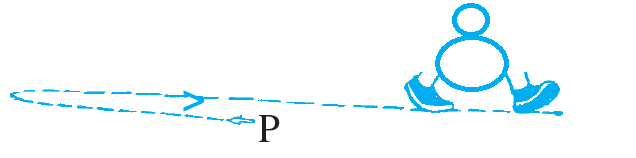# Rational Numbers Practice Test Question Answers [PDF]

 1. Which of the following rational numbers is equivalent to \frac23  ? 🔘 \frac39 🔘 \frac29 🔘 \frac94 🔘 \frac34 🔘 \frac46
 2. Which of the following rational numbers is in standard form? 🔘 \frac12 🔘 \frac{3}{-2} 🔘 \frac{10}{20} 🔘 \frac{-1}{2} 🔘 \frac{10}{4}
 3. The sum of \frac{-3}{2} + \frac{1}{2} 🔘 -3 🔘 -1 🔘 3 🔘 2 🔘 -2
 5.Devid walks \frac23 km from a place P, towards east and then from there 1 \frac57 km towards west. Where will he be now from P?🔘 1\frac{-51}{122} 🔘 1\frac{-120}{7} 🔘 1\frac{1}{121} 🔘 1\frac{120}{7} 🔘 1\frac{51}{2}